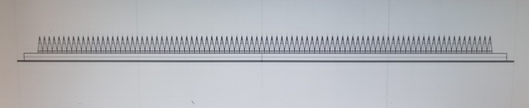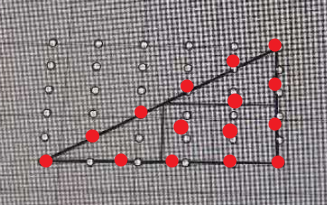# 请教如何脚本实现阵列？1赞

2赞

``````# Creates an array of points on a surface
import rhinoscriptsyntax as rs

def ArrayPointsOnSurface():
# Get the surface object
surface_id = rs.GetObject("Select surface", rs.filter.surface)
if surface_id is None: return

# Get the number of rows
rows = rs.GetInteger("Number of rows", 10, 2)
if rows is None: return

# Get the number of columns
columns = rs.GetInteger("Number of columns", 10, 2)
if columns is None: return

# Get the domain of the surface
U = rs.SurfaceDomain(surface_id, 0)
V = rs.SurfaceDomain(surface_id, 1)
if U is None or V is None: return

for i in xrange(0,rows):
param0 = U + (((U - U) / (rows-1)) * i)
for j in xrange(0,columns):
param1 = V + (((V - V) / (columns-1)) * j)
point = rs.EvaluateSurface(surface_id, param0, param1)

if __name__ == "__main__":
ArrayPointsOnSurface()

``````1赞

``````# Creates an array of points on a surface
import rhinoscriptsyntax as rs

def ArrayPointsOnSurface():
# Get the surface object
surface_id = rs.GetObject("Select surface", rs.filter.surface)
if surface_id is None: return

# Get the number of rows
rows = rs.GetInteger("Number of rows", 10, 2)
if rows is None: return

# Get the number of columns
columns = rs.GetInteger("Number of columns",10, 2)
if columns is None: return

# Get the domain of the surface
U = rs.SurfaceDomain(surface_id, 0)
V = rs.SurfaceDomain(surface_id, 1)
if U is None or V is None: return

for i in xrange(0,rows):
param0 = U + (((U - U) / (rows-1)) * i)
for j in xrange(0,columns):
param1 = V + (((V - V) / (columns-1)) * j)
point = rs.EvaluateSurface(surface_id, param0, param1)
if __name__ == "__main__":
# call the function defined above
ArrayPointsOnSurface()
``````

point in curve的rhinocommon功能对应contains

rhino的wiki关于nurbs曲面的简单介绍